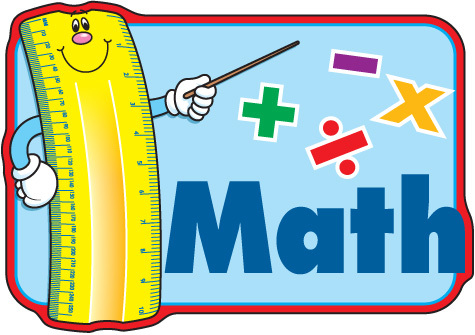4 years ago
mgarcia6
Save
Edit
Host a game
Live GameLive
Homework
Solo Practice
Practice12 QuestionsShow answers
• Question 1
300 seconds
Q. Arrange the following numbers in order from LEAST to GREATEST.2,503  2,305  2,035  2,350
1) 2,503  2) 2,350  3) 2,035  4) 2,305
1)2,035 2) 2,305  3) 2,350  4) 2,503
1) 2,035  2) 2,350  3)2,503  4) 2,305
1) 2, 503  2) 2,305  3) 2,350  4) 2,035
• Question 2
300 seconds
Q. Which number sentence is correct?
A)  32,645 = 32,466
B)  13,946 > 15,999
C)  15,946 < 159,460
D)  4,537 > 6,397
• Question 3
180 seconds
Q. How would the following decimal be read? 0.38
A)  Thirty-eight
B)  Thirty-eight tenths
C)  Thirty-eight hundredths
D)  Thirty-eight thousandths
• Question 4
60 secondsQ. What kind of angles are Angles A, B, C and D?
Right angle
Obtuse angle
Acute angle
Straight angle
• Question 5
60 secondsQ. What kind of lines are these?
Parallel lines
Intersecting lines
Straight lines
Perpendicular lines
• Question 6
60 secondsQ. All triangular prisms have---
8 edges, 6 faces, 6 vertices
9 edges, 5 faces, 6 vertices
6 edges, 9 faces, 5 vertices
5 edges, 6 faces, 8 vertices
• Question 7
60 secondsQ. Which number sentence is represented by the array shown?
4 x 6 = 24
6 x 7 = 42
7 x 4 = 28
4 x 5 = 20
• Question 8
300 secondsQ.
+11, 3 and 8
+9, 1 and 7
+10, 4 and 6
+8, 0 and 4
• Question 9
120 seconds
Q. Which expression is equivalent to 7/8?
1/8 + 1/8 + 1/8  + 1/8
1/7 + 1/8
2/8 + 3/8 + 1/8
1/8 + 1/8 + 1/8 + 4/8
• Question 10
300 seconds
Q. Hailey and Wendy painted an entire wall together. Hailey painted 3/7 of the wall, and Wendy painted the rest. Which statement is true?
A) Hailey painted less than half the wall, and Wendy painted more than half the wall.
B) Hailey painted more than half the wall, and Wendy painted less than half the wall.
C) Each girl painted more than half the wall.
D) Each girl painted less than half the wall.
• Question 11
120 seconds
Q.  Which figure cannot have parallel line segments?
Square
Pentagon
Triangle
Trapezoid
• Question 12
300 seconds
Q. Nathan had a \$3 bill, 2 quarters, 2 dimes, and 4 nickels. He paid for a poster that cost \$3.36. How much money does she have left?